# Highlights

• Self-Attention mechanisms coming from the Transformer layers, but applied to Deep Convolution Auto-Encoder Networks and Generative Adversarial Networks.
• Usage of Fréchet Inception Distance1 as accuracy measure for generated images.

# Introduction

This newly introduce type of GAN is base on networks allowing attention-driven, long-range dependency modelling and applies it to generation tasks. It has been observed that convolutional GANs have much more difficulty in modelling some image classes than other when trained on multi-class datasets. The goal of SAGAN is to allows the network to capture geometric and/or structural patterns that occurs consistently in some classes (for example, generated images of dogs not having defined paws, but they have realistic fur).

## Problems with training convolutional GANs

Despite their great success in various image generation tasks, the training of GANs are known to be unstable and sensitive to the choices of hyper-parameters (extreme dependency) while training. Also, convolutions process information on a local neighborhood, thus loosing the long-range dependencies in images.

## Usage of attention mechanisms

Coming from Attention models, attention mechanisms have become an integral part of models that must capture global dependencies of the data. The authors tried applying this method to a convolutional network.

the image features from the previous hidden layer is $$x \in \mathbb{R}^{C \times N}$$ are first transformed into two feature spaces f,g to calculate the attention, where $$f(x) = W_f\space x$$ and $$g(x) = W_g\space x$$. Then using those variables, they do a softmax on the output such: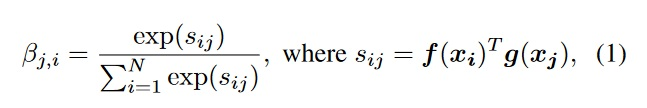to indicate the extent to which the model attends to the $$i^{th}$$ location when synthesizing the j^{th} region.

This sums up to the output of a hidden layer to be $$o = (o_1, o_2, ... o_N) \in R^{C\times N}$$ where: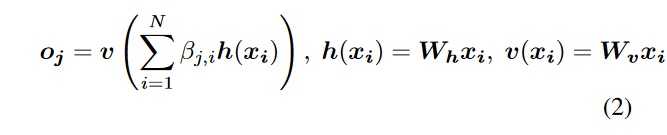All the $$W$$ are learnable weight matrices implemented as $$1 \times 1$$ convolutions. For the $$\beta$$ variable, the channel number is always increase by 8. ($$\bar{C} = C/8$$) for memory optimization (this does not cause performance loss).

Both the discriminant and the generator have the self-attention mechanism applied to them.

## Architecture of a Convolutional layer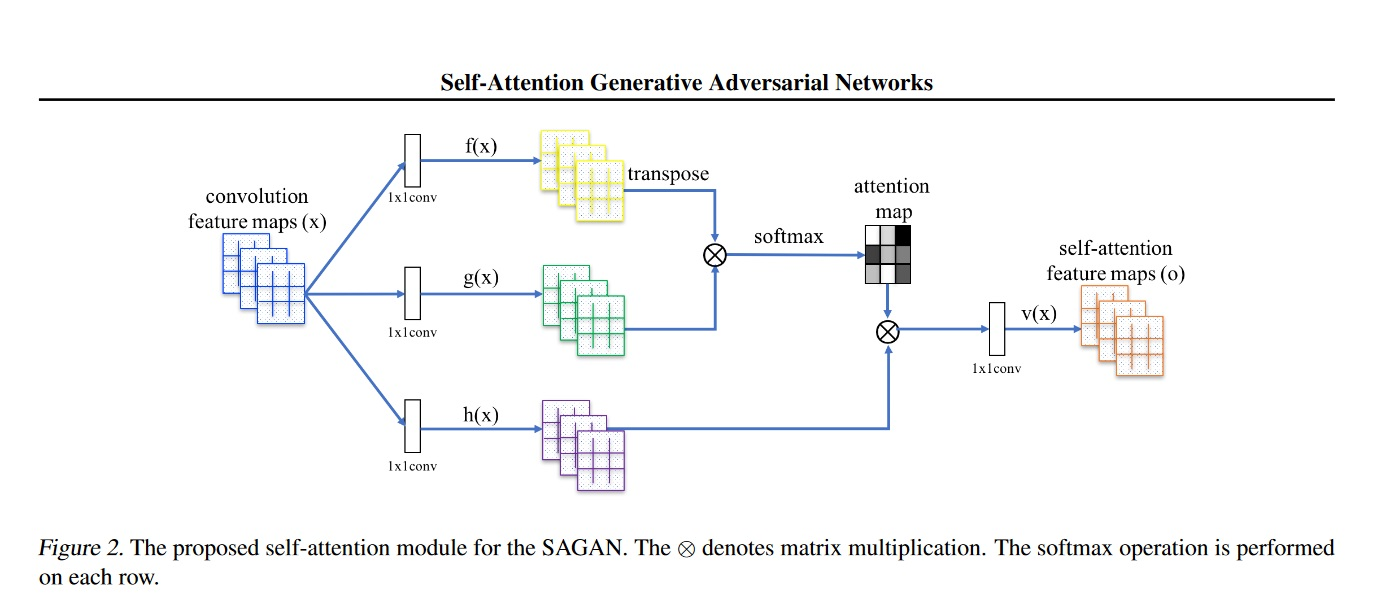## Training methods

• Alternating fashion by minimizing the hinge version of the adversarial loss.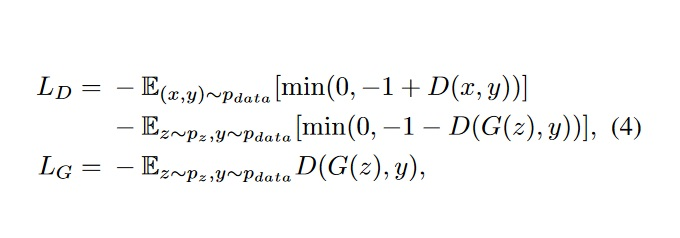• Usage of spectral norm for both Discriminant and Generator
• Imbalanced learning rate for generator and discriminant updates using two time-scale update rule.
• Accuracy measured with
• Fréchet Inception Distance(FID)
• Inception score (KL divergence between conditional class distribution and marginal class distribution)

# Results

It was trained using the LSVRC2012 (ImageNet) dataset. Models were trained for roughly 2 weeks on 4 GPUs each, using synchronous SGD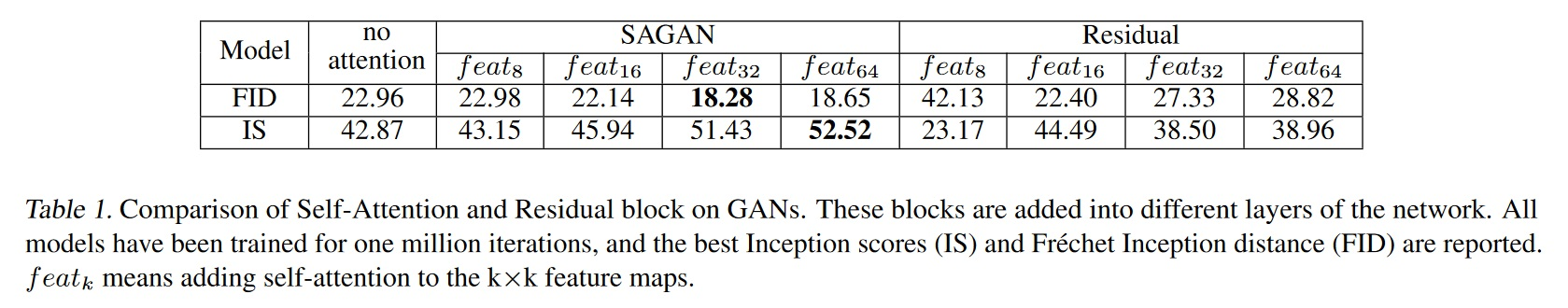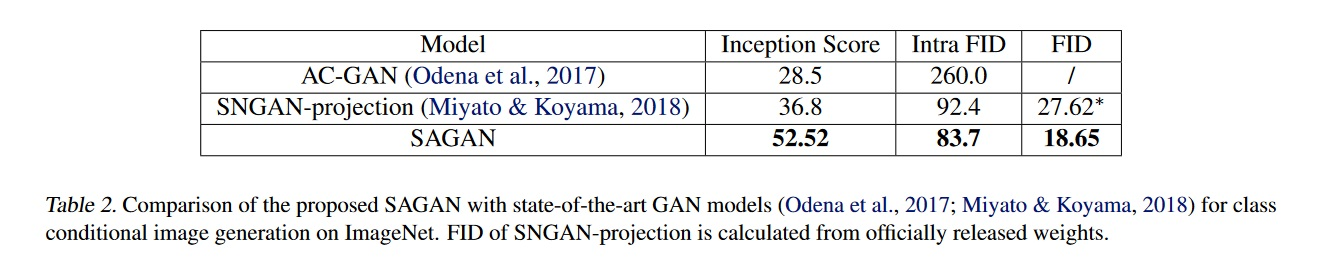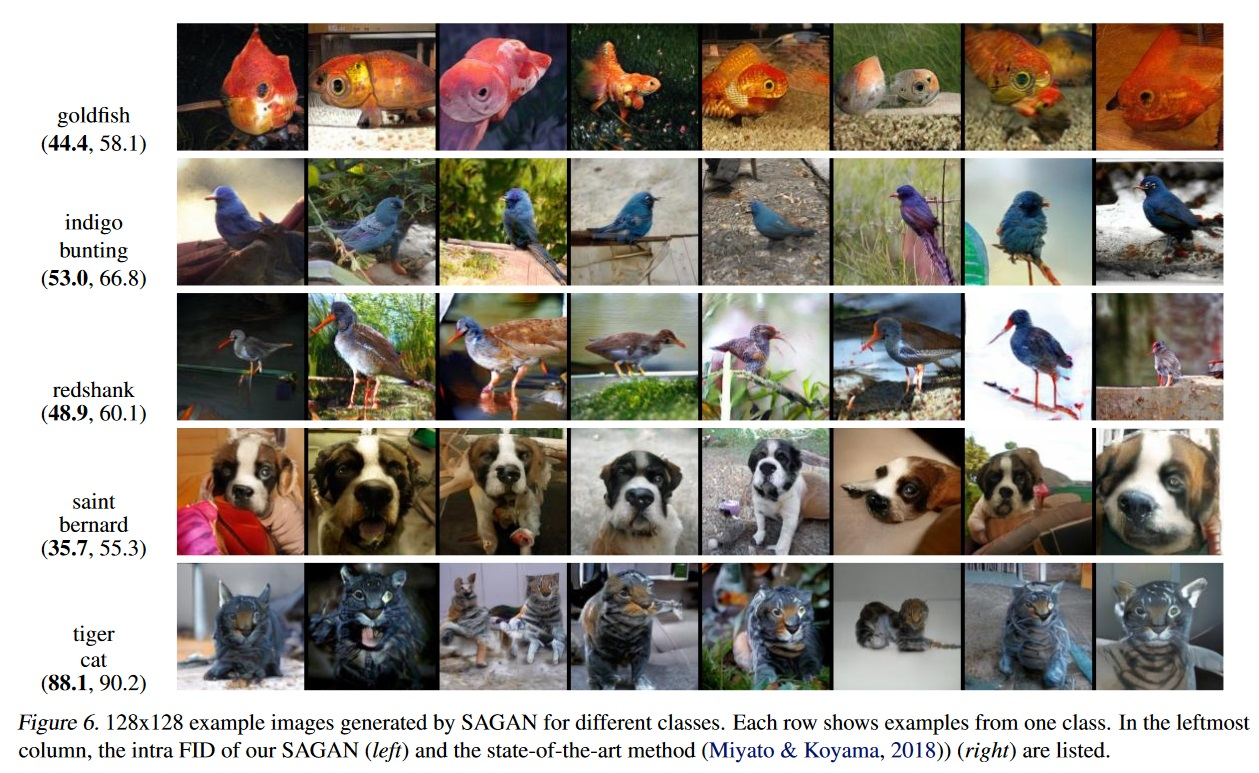# Remarks

• This was taken from the article repository: The current batch size is 64x4=256. Larger batch size seems to give better performance. But it might need to find new hyper parameters for G&D learning rate. Note: It usually takes several weeks to train one million steps.
• There is no mention of how big was the training dataset based on ImageNet. The only thing they mention is that they used LSVRC2012 dataset.
• In the code, the block seems to be bn->relu->upsample->snconv->bn->relu->snconv, then with a copy the input, upsample->snconv for the generator.
1. GANs Trained by a Two Time-Scale Update Rule Converge to a Local Nash Equilibrium (https://arxiv.org/abs/1706.08500)